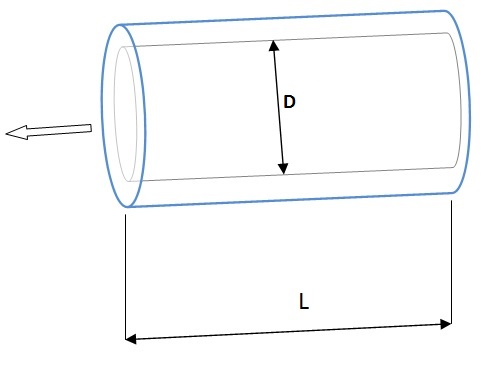# CALCULATE VELOCITY OF THE FLUID FROM FLOW-RATE AND DIAMETER

Enter the flow rate and internal diameter to calculate the velocity of the fluid (length is optional, for lag time calculation).

 Flow rate m3/hl/minl/sft3/minft3/hgal/minUS brl/d Internal diameter (D) mm length (L) -optional- m

The equation used for the calculatin of velocity is:

V [m/s] = Q [m3/h] · (1/3600) / (PI · (D[mm]/2000)^2)